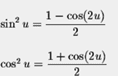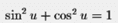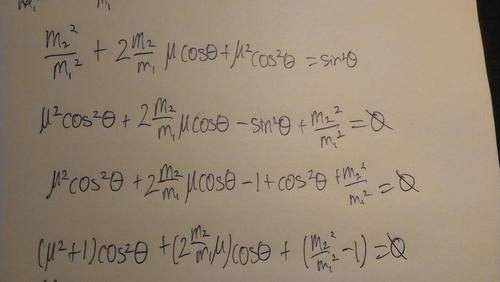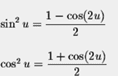# Isolate for Angle With Variables

## Homework Statement

Isolate for the angle. Do not sub in numbers, isolate the angle, θ . Use of trig identities required.

## Homework Equations

$m_{2}g=m_{1}gsinθ-μm_{1}gcosθ$

We are given the trigonometric identities:## The Attempt at a Solution

I have attempted everything from squaring both sides, to no avail, as I see no way to use any trig identities so isolate for the angle. It is the μ that makes it quite difficult to isolate for θ.

$m_{2}g=m_{1}gsinθ-μm_{1}gcosθ$
$m_{2}g=m_{1}g(sinθ-μcosθ)$
$\frac{m_{2}g}{m_{1}g}=(sinθ-μcosθ)$
$\frac{m_{2}}{m_{1}}=sinθ-μcosθ$
At this point I am completely stuck... Any help would be appreciated.

Thanks.

Last edited:

nasu
Are you trying to solve for the angle in therm of the other variables?

•1 person
Yes, that is what I am trying to do.

nasu
You could move one of the terms from right side to left and then square both sides.
Then use the last of the formulas in your image to get a quadratic equation in either sin or cos.

•1 person
Alright. This is what I have tried... I don't know if it's entirely correct but it still seems really clustered.I don't know what to do next though...

Mentor

## Homework Statement

Isolate for the angle. Do not sub in numbers, isolate the angle, θ . Use of trig identities required.

## Homework Equations

$m_{2}g=m_{1}gsinθ-μm_{1}gcosθ$

We are given the trigonometric identities:## The Attempt at a Solution

I have attempted everything from squaring both sides, to no avail, as I see no way to use any trig identities so isolate for the angle. It is the μ that makes it quite difficult to isolate for θ.

$m_{2}g=m_{1}gsinθ-μm_{1}gcosθ$
$m_{2}g=m_{1}g(sinθ-μcosθ)$
$\frac{m_{2}g}{m_{1}g}=(sinθ-μcosθ)$
$\frac{m_{2}}{m_{1}}=sinθ-μcosθ$
At this point I am completely stuck... Any help would be appreciated.

Thanks.
Try this:
$(sinθ-μcosθ)=\sqrt{μ^2+1}(\frac{1}{\sqrt{μ^2+1}}sinθ-\frac{μ}{\sqrt{μ^2+1}}cosθ)=\sqrt{μ^2+1}(sinθcos\phi-cosθsin\phi)=\sqrt{μ^2+1}\,\,sin(θ-\phi)$
where $cot\phi=μ$

Homework Helper
Alright. This is what I have tried... I don't know if it's entirely correct but it still seems really clustered.I don't know what to do next though...

You got a quadratic equation for cosθ. Solve with the quadratic formula.

ehild

Hi, just attempted with quadratic formula, but I can't get anywhere (as in I can't simplify it any further it looks so hideous).

Mentor
Since no one seemed to have any interest in what I did in response #6, I will continue by completing the solution:

$$θ=\arcsin\left({\frac{(m_2/m_1)}{\sqrt{μ^2+1}}}\right)+\arctan(1/μ)$$

•1 person
nasu
Since no one seemed to have any interest in what I did in response #6, I will continue by completing the solution:

$$θ=\arcsin\left({\frac{(m_2/m_1)}{\sqrt{μ^2+1}}}\right)+\arctan(1/μ)$$

This is a clever method. You can even take μ=tan(β) where β is the "friction angle", which has some physical interpretation.

Edit. Actually the ∅ defined as above it is equal to the friction angle:
tan(∅)=μ.

Are you sure that in your result, the last term is not arctan(μ) rather than of 1/μ?

Last edited:
Homework Helper
Hi, just attempted with quadratic formula, but I can't get anywhere (as in I can't simplify it any further it looks so hideous).

You can simplify it a bit, by expanding the parentheses under the square root. And don't worry if it is not simple.

ehild

Homework Helper
Since no one seemed to have any interest in what I did in response #6, I will continue by completing the solution:

$$θ=\arcsin\left({\frac{(m_2/m_1)}{\sqrt{μ^2+1}}}\right)+\arctan(1/μ)$$

It should be arctan(μ).
Yes, it is a clever method if somebody knows it.

ehild

Mentor
It should be arctan(μ).
Yes, it is a clever method if somebody knows it.

ehild

Oh. You're right. It should be arctan(μ). Sorry for the error.
Incidentally, this "clever method" has appeared in many threads on physics forums. It is often used to get the phase angle in periodic solutions to problems.

Homework Helper
Incidentally, this "clever method" has appeared in many threads on physics forums.

The OP did not know it, so some explanation would have been necessary.

ehild

nasu
Oh. You're right. It should be arctan(μ). Sorry for the error.
Incidentally, this "clever method" has appeared in many threads on physics forums. It is often used to get the phase angle in periodic solutions to problems.

I did not say it's new. We used in high school and that was long time ago.But this does not make it less clever, right? Or at least more elegant that the quadratic equation.

However, as ehild pointed already a couple of times, the poster may not be aware of the trig identities involved. The less elegant methods may feel more comfortable for a beginner.

Mentor
The OP did not know it, so some explanation would have been necessary.

ehild

Good point. I guess a couple of extra details would have been really helpful. I'll try to remember this for the future.

Chet